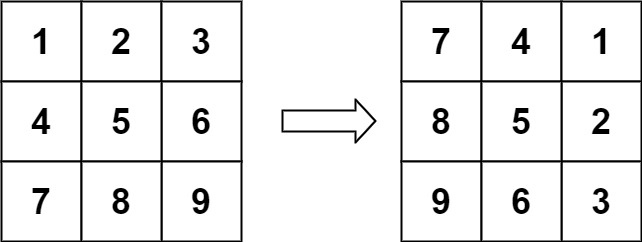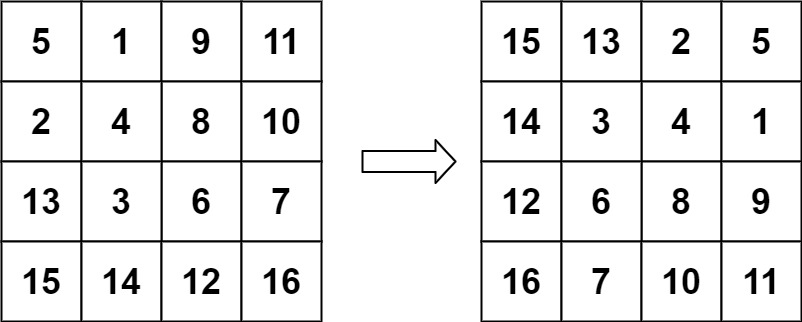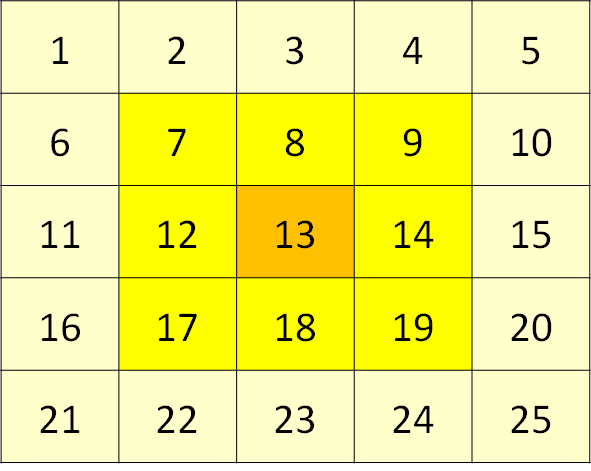# Rotate Image

## 1: U-nderstand

Understand what the interviewer is asking for by using test cases and questions about the problem.

• Established a set (2-3) of test cases to verify their own solution later.
• Established a set (1-2) of edge cases to verify their solution handles complexities.
• Have fully understood the problem and have no clarifying questions.
• Have you verified any Time/Space Constraints for this problem?
• Can the input grid be blank??
• Let’s assume the grid is not blank. We don’t need to consider empty inputs.
• Can the row size be different from the column size?
• No, the row size is equal to the column.
• What are the time and space constraints?
• Time complexity should be `O(m*n)`, m being the rows of the matrix and n being the columns of matrix. Space complexity should be `O(1)`.
``````HAPPY CASE
Input: matrix = [[1,2,3],[4,5,6],[7,8,9]]
Output: [[7,4,1],[8,5,2],[9,6,3]]````````````Input: matrix = [[5,1,9,11],[2,4,8,10],[13,3,6,7],[15,14,12,16]]
Output: [[15,13,2,5],[14,3,4,1],[12,6,8,9],[16,7,10,11]]
````````````EDGE CASE
Input: matrix = []
Output: []``````

## 2: M-atch

Match what this problem looks like to known categories of problems, e.g. Linked List or Dynamic Programming, and strategies or patterns in those categories.

For 2D-Array, common solution patterns include:

• Perform a BFS/DFS Search through the 2D Array
• A search through the 2D Array (either BFS or DFS) does not help us. We are rotating an image.
• Hash the 2D Array in some way to help with the Strings
• Hashing would not help us flipping a image horizontally, then inverting it
• Create/Utilize a Trie
• A Trie would not help us much in this problem since we are not trying to determine anything about a sequence of characters.

## 3: P-lan

Plan the solution with appropriate visualizations and pseudocode.

General Idea: Let's recursively rotate each item layer by layer.

``````1) Create a helper function with the boundary for rotation
a)Basecase: Single square
b)Rotate the items from upper-row with left-column, left-column with bottom-row, bottom-row with right-column, and right-column with upper-row
i) Store upper-row
ii) Set upper-row with value in left-column
iii) Set left-column with value in bottom-row
iv) Set bottom-row with value in right-column
v) Set the right-column with upper-row
c)Recursive reduce the layers
2) Run helper function starting at the outermost layer``````⚠️ Common Mistakes

• Not every 2D-Array problem follows the common techniques.

## 4: I-mplement

Implement the code to solve the algorithm.

``````class Solution:
def rotate(self, matrix: List[List[int]]) -> None:
"""
Do not return anything, modify matrix in-place instead.
"""
def helper(top_left, bottom_right):
# Basecase: Single square
if bottom_right <= top_left:
return

# Rotate the items from upper-row with left-column, left-column with bottom-row, bottom-row with right-column, and right-column with upper-row
for i in range(top_left, bottom_right):
tmp = matrix[top_left][i] # Store upper-row
matrix[top_left][i] = matrix[n-i][top_left] # Set upper-row with value in left-column
matrix[n-i][top_left] = matrix[n-top_left][n-i] # Set left-column with value in bottom-row
matrix[n-top_left][n-i] = matrix[n-(n-i)][n-top_left] # Set bottom-row with value in right-column
matrix[n-(n-i)][n-top_left] = tmp # Set the right-column with upper-row

# Recursive reduce the layers
helper(top_left+1, bottom_right-1)

# Run helper function starting at the outermost layer
n = len(matrix)-1
helper(0, n)``````
``````class Solution {
public void rotate(int[][] matrix) {
// Run helper function starting at the outermost layer
int n = matrix.length;
solve(matrix, n, 0);
return;
}

void solve(int[][] matrix, int n, int start){
// Basecase: Center square
if(start >= n/2){
return;
}

// Rotate the items from upper-row with left-column, left-column with bottom-row, bottom-row with right-column, and right-column with upper-row
for(int i = start; i < n-1-start; i++){
int a = matrix[start][i];
int b = matrix[i][n-1-start];
int c = matrix[n-1-start][n-i-1];
int d = matrix[n-i-1][start];

matrix[start][i] = d;
matrix[i][n-1-start] = a;
matrix[n-1-start][n-i-1] = b;
matrix[n-i-1][start] = c;
}

// Recursive reduce the layers
solve(matrix, n, start+1);
}
}``````

## 5: R-eview

Review the code by running specific example(s) and recording values (watchlist) of your code's variables along the way.

• Trace through your code with an input to check for the expected output
• Catch possible edge cases and off-by-one errors

## 6: E-valuate

Evaluate the performance of your algorithm and state any strong/weak or future potential work.

Assume `N` represents the number of rows in 2D-array. Assume `M` represents the number of columns in 2D-array.

• Time Complexity: O(N * M) we need to rotate each item in the 2D-Array
• Space Complexity: O(1), because we are not using any additional space, we rotate the image in-place.